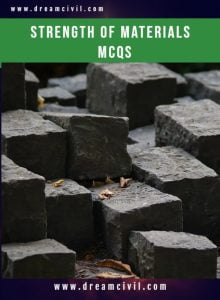Monday, September 25, 2023

Strength of Materials Objective Questions ( MCQs ) PDF containing 328 questions is given below. You can directly download this PDF and study from it.

### 1. Details of the PDFTopic of MCQs Strength of Materials Size 539 KB Pages 66 Number of Questions 328

### 2. Some questions from PDF

Question No. 1
The Rankine-Golden formula accounts for direct as well as buckling stress and is applicable to

(A) Very long columns
(B) Long columns
(C) Short columns
(D) Intermediate columns

Question No. 2
The neutral axis of a beam cross-section must

(A) Pass through the centroid of the section
(B) Be equidistant from the top of bottom films
(C) Be an axis of symmetry of the section
(D) None of these

Question No. 3
In a continuous bending moment curve, the point where it changes sign is called

(A) Point of inflexion
(B) Point of contraflexure
(C) Point of the virtual hinge
(D) All the above

Question No. 4
The equivalent length of a column fixed at one end and free at the other end, is

(A) 0.5 l
(B) 0.7 l
(C) 2 l
(D) 1.5 l

Question No. 5
For a given material, if E, C, K and m are Young’s modulus, shearing modulus, bulk modulus and Poisson ratio, the following relation does not hold good

(A) E = 9KC/3K + C
(B) E = 2K (1 + 2/m)
(C) E = 2C (1 + 1/m)
(D) E = 3C (1 – 1/m)

Question No. 6
A short masonry pillar is 60 cm x 60 cm in cross-section, the core of the pillar is a square whose side is

(A) 17.32 cm
(B) 14.14 cm
(C) 20.00 cm
(D) 22.36 cm

Question No. 7
The ratio of the maximum deflection of a cantilever beam with an isolated load at its free end and with a uniformly distributed load over its entire length is

(A) 1
(B) 24/15
(C) 3/8
(D) 8/3

Question No. 8
The slenderness ratio of a vertical column of a square cross-section of 10 cm wide and 500 cm long, is

(A) 117.2
(B) 17.3
(C) 173.2
(D) 137.2

Question No. 9
A steel rod of 2 cm diameter and 5 metres long is subjected to an axial pull of 3000 kg. If E = 2.1 × 10^6, the elongation of the rod will be

(A) 2.275 mm
(B) 0.2275 mm
(C) 0.02275 mm
(D) 2.02275 mm

Question No. 10
A rectangular log of wood is floating in water with a load of 100 N at its centre. The maximum shear force in the wooden log is

(A) 50 N at each end
(B) 50 N at the centre
(C) 100 N at the centre
(D) None of these

Question No. 11
The maximum compressive stress at the top of a beam is 1600 kg/cm² and the corresponding tensile stress at its bottom is 400 kg/cm². If the depth of the beam is 10 cm, the neutral axis from the top, is

(A) 2 cm
(B) 4 cm
(C) 6 cm
(D) 8 cm

Question No. 12
At either end of a plane frame, the maximum number of possible transverse shear forces, are

(A) One
(B) Two
(C) Three
(D) Four

Question No. 13
In a square beam loaded longitudinally, shear develops

(A) On middle fibre along the horizontal plane
(B) On lower fibre along the horizontal plane
(C) On top fibre along the vertical plane
(D) Equally on each fibre along the horizontal plane

Question No. 14
Reactions at the supports of a structure can be determined by equating the algebraic sum of

(A) Horizontal forces to zero
(B) Vertical forces to zero
(C) Moment about any point to zero
(D) All the above

Question No. 15
The phenomenon of the slow growth of strain under steady tensile stress is called

(A) Yielding
(B) Creeping
(C) Breaking
(D) None of these

Question No. 16
If the stress in each cross-section of a pillar is equal to its working stress, it is called

(A) Body of equal
(B) Body of equal section
(C) Body of equal strength
(D) None of these

Question No. 17
The maximum deflection of a cantilever due to pure bending moment M at its free end is

(A) ML²/3EI
(B) ML²/4EI
(C) ML²/6EI
(D) ML²/2EI

Thank you ! for visiting us. If you have any queries then kindly mail us at madhukrishnapoudel151@gmail.com or WhatsApp us at 9804406747.He is a founder and lead author of Dream Civil International and his civil engineering research articles has been taken as source by world's top news and educational sites like USA Today, Time, The richest, Wikipedia, etc.
Latest Articles

Related Articles